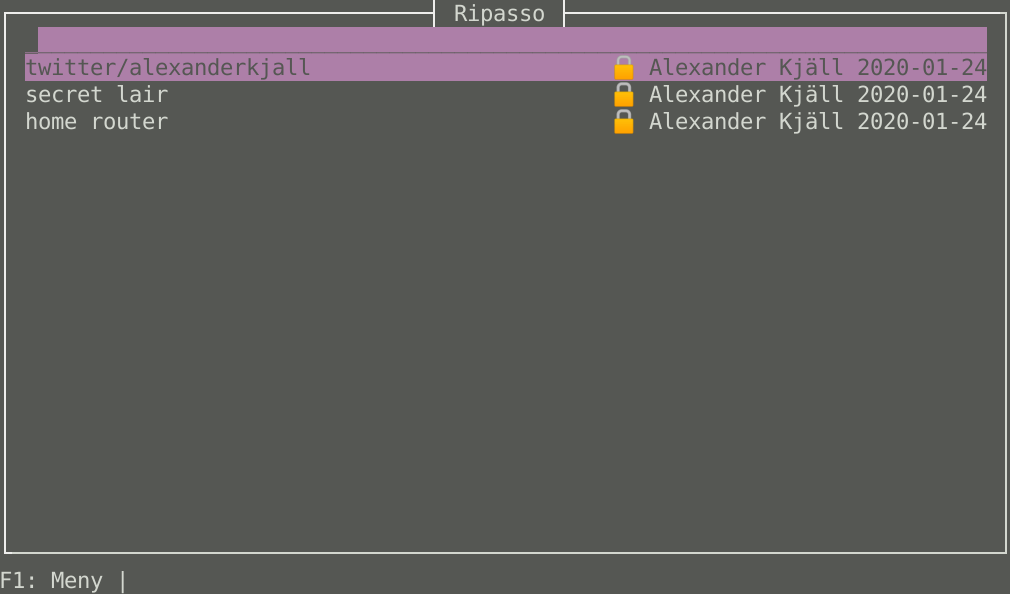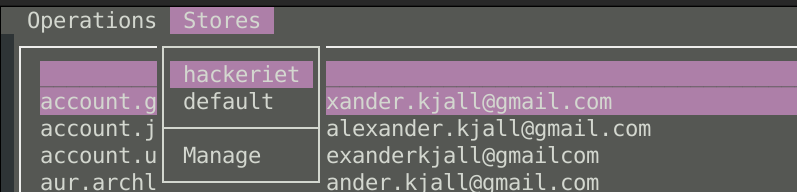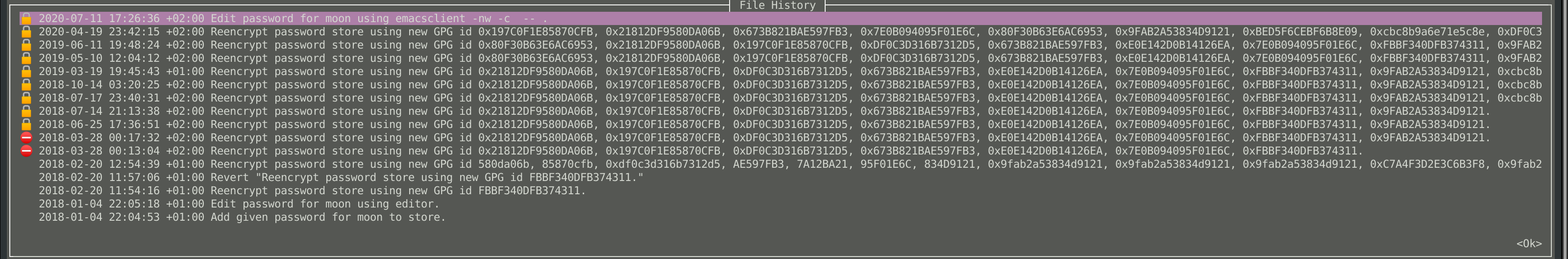# Release of Ripasso version 0.5.0After nine long months of development effort, we are proud to present ripasso version 0.5.0.

## New Features

We have implemented support for configuration files. You can now switch between different password directories from the menu.#### Fuzzing of our dependencies

We did a small project where we went over our dependencies with a fuzzer, bugs found:

Some of them have been closed, some are in optional dependencies that we now have excluded and some are in a package that we want to start using in the future.

If you press ctrl-H on a password entry, it will bring up the git history of that file.Copy the file name with ctrl-U, this can be useful if you have your username as the filename.

## Bugs Fixed

#### Passwords in initial commit causes error

If the initial git commit contained files, that caused errors as ripasso didn’t consider that snapshot correctly.

#### Not assume that git branch should be named master

A hardcoding of the branch name was removed.

## Credits

• Joakim Lundborg - Developer
• Alexander Kjäll - Developer
• Silje Enge Kristensen - Norwegian bokmål translation
• Camille Victor Prunier - French translation
• David Plassmann - German translation

Also a big thanks to everyone who contributed with bug reports and patches.

# Solution to Bornhack 2020 CTF challenge nc333nc333

crypto

50

#### Writeup

This was the second challenge in the crypto category, this time with a lot of different encodings.

We got this file that contained a large number of encoded strings nested inside each other like a russian doll.

We wrote a small rust program to handle it:

``````use std::fs::File;
use std::io::prelude::*;

use base64::decode;
use crate::Commands::{Base64, Reverse, Rot13, Hex};

enum Commands {
Base64,
Reverse,
Rot13,
Hex
}

impl Commands {
fn from(s: &str) -> Option<Commands> {
if s.eq("base64") {
return Some(Base64);
}
if s.eq("reverse") {
return Some(Reverse);
}
if s.eq("rot13") {
return Some(Rot13);
}
if s.eq("hex") {
return Some(Hex)
}

eprintln!("unknown command: {}", s);
None
}
}

fn split_once(in_string: &str) -> (&str, &str) {
let mut splitter = in_string.splitn(2, ':');
let first = splitter.next().unwrap();
let second = splitter.next().unwrap();
(first, second)
}

fn rot(c :&char) -> char {
if c.is_ascii_alphabetic() {
let a = if c.is_ascii_lowercase() {
b'a'
} else {
b'A'
};
let mut utf8 = [0u8; 1];
c.encode_utf8(&mut utf8);
let rot = (((utf8 - a) + 13) % 26) + a;
std::char::from_u32(rot as u32).unwrap()
} else {
*c
}
}

fn rot13(s: &String) -> String {
s.chars().map(|c| rot(&c)).collect()
}

fn main() -> std::io::Result<()> {
let mut file = File::open("challenge")?;
let mut contents = String::new();

while !contents.starts_with("BHCTF") {
let (command, text) = split_once(&contents);
let command = Commands::from(command).unwrap();
let decoded = match command {
Base64 => {
let t = &decode(text).unwrap();
std::str::from_utf8(t).unwrap().to_string()
},
Reverse => text.chars().rev().collect::<String>(),
Rot13 => rot13(&text.to_string()),
Hex => {
let t = hex::decode(text).unwrap();
std::str::from_utf8(&t).unwrap().to_string()
}
};
contents = decoded;
}
println!("{}", contents);
Ok(())
}
``````

Flag was `BHCTF{b4se64_is_n0t_crypt0}`.

# Solution to Bornhack 2020 CTF challenge nc3nc3

crypto

25

#### Writeup

This was the first challenge in the crypto category, just a couple of different encodings.

Challenge text:

``````base64:cmV2ZXJzZTo5UldZaTkxYno5RmR1TlhZMzlGZGhoR2Q3WkVWRGhrUTo0NmVzYWI=
``````

We solved it with bash:

``````#!/bin/bash

cat nc3 | awk '{ print(substr(\$0, 8, length(\$0)))}'|base64 -d |\
awk '{ print(substr(\$0, 9, length(\$0)))}'|rev|\
awk '{ print(substr(\$0, 8, length(\$0)))}'|base64 -d
echo
``````

Flag was `BHCTF{that_wasnt_so_bad}`.

# Solution to Bornhack 2020 CTF challenge caesar_with_a_twist##### Name:

caesar_with_a_twist

crypto

75

#### Writeup

The third challenge in the crypto category, this was the first one with some actual encryption.

We got an encrypted text:

``````CLLJE{QtLo_wF_r_YqOI_bXrH_gpJw_WxPh_Sm_QraVmo_Se_IoSvvn_XyR_GeU_Ack_EDRDTIosscf_rj}
``````

And a Python program that described how it was generated, analysing that program showed that it was a regular substitution cipher where the substitution key was changed for each character. The key was a function of that characters position in the string.

We reimplemented it in rust and wrote a reverse implementation:

``````use std::fs::File;
use std::io::prelude::*;

#[derive(Debug, Clone)]
struct CaesarError;

fn caesar_encrypt(original: char, base: u32) -> Result<char, CaesarError> {
let letter = original as u32;

// Ensures the base rotation does not exceed 26
// If the base is 28, it exceeds 26, and ends up being 2 (after modulus)
let base = base % 26;

// If the letter is a space, underscore or curly brackets, just return it without rotating
if letter == 32 || letter == 95 || letter == 123 || letter == 125 {
return Ok(std::char::from_u32(letter).unwrap());
}

// If capital letter
if 'A' as u32 <= letter && letter <= 'Z' as u32 {
// If the base exceeds the alphabet
return if ('Z' as u32) < (letter + base) {
Ok(std::char::from_u32(letter + base - 26).unwrap())
} else {
Ok(std::char::from_u32(letter + base).unwrap())
}
}

// If non-capital letter
if 'a' as u32 <= letter && letter <= 'z' as u32 {
// If the base exceeds the alphabet
return if ('z' as u32) < (letter + base) {
Ok(std::char::from_u32(letter + base - 26).unwrap())
} else {
Ok(std::char::from_u32(letter + base).unwrap())
}
}

Err(CaesarError {})
}

fn caesar_decrypt(original: char, base: u32) -> Result<char, CaesarError> {
let letter = original as u32;

// Ensures the base rotation does not exceed 26
// If the base is 28, it exceeds 26, and ends up being 2 (after modulus)
let base = base % 26;

// If the letter is a space, underscore or curly brackets, just return it without rotating
if letter == 32 || letter == 95 || letter == 123 || letter == 125 {
return Ok(std::char::from_u32(letter).unwrap());
}

// If capital letter
if 'A' as u32 <= letter && letter <= 'Z' as u32 {
// If the base exceeds the alphabet
return if ('A' as u32) > (letter - base) {
Ok(std::char::from_u32(letter - base + 26).unwrap())
} else {
Ok(std::char::from_u32(letter - base).unwrap())
}
}

// If non-capital letter
if 'a' as u32 <= letter && letter <= 'z' as u32 {
// If the base exceeds the alphabet
return if ('a' as u32) > (letter - base) {
Ok(std::char::from_u32(letter - base + 26).unwrap())
} else {
Ok(std::char::from_u32(letter - base).unwrap())
}
}

Err(CaesarError {})
}

fn encrypt(s: &String) -> Result<String, CaesarError> {
let mut result:Vec<char> = vec![];
for (i, c) in s.chars().enumerate() {
result.push(caesar_encrypt(c, ((i + 1) * (i + 1)) as u32)?);
}
Ok(result.iter().collect::<String>())
}

fn decrypt(s: &String) -> Result<String, CaesarError> {
let mut result:Vec<char> = vec![];
for (i, c) in s.chars().enumerate() {
result.push(caesar_decrypt(c, ((i + 1) * (i + 1)) as u32)?);
}
Ok(result.iter().collect::<String>())
}

fn main() -> std::io::Result<()> {
let mut file = File::open("cwat_encrypted_flag.txt")?;
let mut contents = String::new();

println!("{}", decrypt(&contents).unwrap());
Ok(())
}

#[cfg(test)]
mod tests {
use crate::CaesarError;
use crate::caesar_decrypt;
use crate::caesar_encrypt;
use crate::encrypt;
use crate::decrypt;

static ASCII_LOWER: [char; 26] = [
'a', 'b', 'c', 'd', 'e',
'f', 'g', 'h', 'i', 'j',
'k', 'l', 'm', 'n', 'o',
'p', 'q', 'r', 's', 't',
'u', 'v', 'w', 'x', 'y',
'z',
];

static ASCII_UPPER: [char; 26] = [
'A', 'B', 'C', 'D', 'E',
'F', 'G', 'H', 'I', 'J',
'K', 'L', 'M', 'N', 'O',
'P', 'Q', 'R', 'S', 'T',
'U', 'V', 'W', 'X', 'Y',
'Z',
];

#[test]
fn loop_all_ascii_chars() -> Result<(), CaesarError> {
for base in 0..25 {
println!("base = {}", base);
for c in &ASCII_LOWER {
assert_eq!(*c, caesar_decrypt(caesar_encrypt(*c, base)?, base)?);
}
for c in &ASCII_UPPER {
assert_eq!(*c, caesar_decrypt(caesar_encrypt(*c, base)?, base)?);
}
}

Ok(())
}

#[test]
fn encrypt_test() -> Result<(), CaesarError> {
assert_eq!("Ulri sp d ksfh", encrypt(&"This is a test".to_string())?);

Ok(())
}

#[test]
fn encrypt_loop() -> Result<(), CaesarError> {
assert_eq!("This is a test", decrypt(&encrypt(&"This is a test".to_string())?)?);

Ok(())
}
}
``````

Flag was `BHCTF{ThIs_iS_a_VeRY_lOnG_flAg_MaDe_By_CaeSar_To_EnSure_YoU_DiD_Not_BRUTUSforce_it}`.

# Solution to Bornhack 2020 CTF challenge alice_bob_playing_telepathy##### Name:

alice_bob_playing_telepathy

crypto

400

#### Writeup

This was a challenge centered around attacking the Lua runtime in a C program.

We got this program

``````#include <stdlib.h>
#include <stdio.h>
#include <unistd.h>
#include <sys/types.h>
#include <sys/stat.h>
#include <fcntl.h>

#include <lauxlib.h>
#include <lua.h>
#include <lualib.h>
#include <string.h>

#include <assert.h>

ssize_t readn(int fd, char *buf, size_t n){
size_t off = 0;
while(off < n){
ssize_t res = read(fd, &buf[off], n-off);
if(res <= 0) return res;
off += res;
}
return off;
}

int main(int argc, char **argv){
alarm(30);

setbuf(stdin, NULL);
setbuf(stdout, NULL);
setbuf(stderr, NULL);
puts("Give me some Alice and Bob:");

char program[256 + 1] = {0};

lua_State *alice = luaL_newstate();
//luaL_openlibs(alice); is too powerful
luaL_requiref(alice, "math", luaopen_math, 1);
luaL_requiref(alice, "table", luaopen_table, 1);
luaL_dostring(alice, program);

lua_State *bob = luaL_newstate();
//luaL_openlibs(bob); is too powerful
luaL_requiref(bob, "math", luaopen_math, 1);
luaL_requiref(bob, "table", luaopen_table, 1);
luaL_dostring(bob, program);

int urandom = open("/dev/urandom", O_RDONLY);
assert(urandom > 0);

for(int i = 0; i < 1000; i++){
unsigned int num = 0;
assert(readn(urandom, (char *)&num, sizeof(num)) == sizeof(num));
num = num % 100;

// alice(num)
lua_getglobal(alice, "alice");
lua_pushnumber(alice, (float)num);
assert(lua_pcall(alice, 1, 0, 0) == 0);

// assert(num == bob())
lua_getglobal(bob, "bob");
assert(lua_pcall(bob, 0, 1, 0) == 0);
assert(lua_isnumber(bob, -1));
assert(num == (int)lua_tonumber(bob, -1));
lua_pop(bob, 1);
}

lua_close(alice);
lua_close(bob);

puts(
#include "flag.h"
);

}
``````

It initializes two different `lua_State` objects from the same source code that the attacker supplies. Source that should have one function named `alice` that accepts a parameter, and another function `bob` that should return a number.

It then generates a series of 1000 random numbers between 0 and 100. Each of those gets sent to the `alice` function in the first `lua_State` and a number is read from the `bob` function in the second `lua_State`. Those two number are verified to be equal.

This means that we need to communicate between functions in different `lua_State`.

That the lua math module is loaded gave us a hint, and the random number generator seed turns out to not be part of the `lua_State` object in lua 5.3 and below, this security hole has been fixed in 5.4.

We wrote this function to reveal the relationship between the `randomseed()` and the `random()` function.

``````
function alice(n)
math.randomseed(0)
for i=0,n do
math.random()
end
end
function bob()
a = math.random()
math.randomseed(0)
for i=0,101 do
if math.random() == a then
return i-1
end
end
end
``````

Sadly the CTF closed just before we sent this in, so we didn’t manage to get the flag, and could only verify this locally.